SSC CGL Previous Year Questions: Mensuration - 3

# SSC CGL Previous Year Questions: Mensuration - 3 - SSC CGL

Test Description

## 40 Questions MCQ Test SSC CGL (Tier - 1) - Previous Year Papers (Topic Wise) - SSC CGL Previous Year Questions: Mensuration - 3

SSC CGL Previous Year Questions: Mensuration - 3 for SSC CGL 2023 is part of SSC CGL (Tier - 1) - Previous Year Papers (Topic Wise) preparation. The SSC CGL Previous Year Questions: Mensuration - 3 questions and answers have been prepared according to the SSC CGL exam syllabus.The SSC CGL Previous Year Questions: Mensuration - 3 MCQs are made for SSC CGL 2023 Exam. Find important definitions, questions, notes, meanings, examples, exercises, MCQs and online tests for SSC CGL Previous Year Questions: Mensuration - 3 below.
Solutions of SSC CGL Previous Year Questions: Mensuration - 3 questions in English are available as part of our SSC CGL (Tier - 1) - Previous Year Papers (Topic Wise) for SSC CGL & SSC CGL Previous Year Questions: Mensuration - 3 solutions in Hindi for SSC CGL (Tier - 1) - Previous Year Papers (Topic Wise) course. Download more important topics, notes, lectures and mock test series for SSC CGL Exam by signing up for free. Attempt SSC CGL Previous Year Questions: Mensuration - 3 | 40 questions in 40 minutes | Mock test for SSC CGL preparation | Free important questions MCQ to study SSC CGL (Tier - 1) - Previous Year Papers (Topic Wise) for SSC CGL Exam | Download free PDF with solutions
 1 Crore+ students have signed up on EduRev. Have you?
SSC CGL Previous Year Questions: Mensuration - 3 - Question 1

### A flask in the shape of a right circular cone of height 24 cm is filled with water. The water is poured in a right circular cylindrical flask whose radius is 1rd/3 of the radius of the base of the circular cone. Then the height of the water in the cylindrical flask is      (SSC CHSL 2014)

Detailed Solution for SSC CGL Previous Year Questions: Mensuration - 3 - Question 1

Let radius of base of cone be r and height of cylinder be h.
Vol. of cone = Vol. of cylinder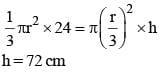SSC CGL Previous Year Questions: Mensuration - 3 - Question 2

### If the sum of the dimensions of a rectangular parallelepiped is 24 cm and the length of the diagonal is 15 cm, then the total surface area of it is   (SSC CHSL 2014)

Detailed Solution for SSC CGL Previous Year Questions: Mensuration - 3 - Question 2

Let length, breadth and height of parallelopiped be l, b and h respectively.
l + b + h = 24 cm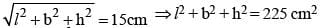(l + b + h)2 – 2(lb + hb + lh) = 225
(24)2 – 225 = 2(lb + bh + hl)
351 = 2 (lb + bh + hl)
Total surface area is 351 cm2.

SSC CGL Previous Year Questions: Mensuration - 3 - Question 3

### Area of a regular hexagon with side 'a' is   (SSC CHSL 2014)

Detailed Solution for SSC CGL Previous Year Questions: Mensuration - 3 - Question 3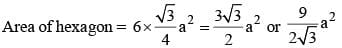SSC CGL Previous Year Questions: Mensuration - 3 - Question 4

A sphere is placed inside a right circular cylinder so as to touch the top, base and the lateral surface of the cylinder. If the radius of the sphere is R, the volume of the cylinder is   (SSC Sub. Ins. 2014)

Detailed Solution for SSC CGL Previous Year Questions: Mensuration - 3 - Question 4

Height of cylinder = 2R
Volume of cylinder = πR2 x (2R) = 2πR3

SSC CGL Previous Year Questions: Mensuration - 3 - Question 5

A rectangular piece of paper of dimensions 22 cm by 12 cm is rolled along its length to form a cylinder. The volume (in cm3) of the cylinder so formed is (use π = 22/7)    (SSC Sub. Ins. 2014)

Detailed Solution for SSC CGL Previous Year Questions: Mensuration - 3 - Question 5

2πr = 22 cm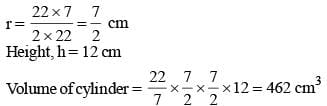SSC CGL Previous Year Questions: Mensuration - 3 - Question 6

Two circular cylinders of equal volume have their heights in the ratio 1 : 2; Ratio of their radii is (Take π = 22/7)    (SSC Sub. Ins. 2014)

Detailed Solution for SSC CGL Previous Year Questions: Mensuration - 3 - Question 6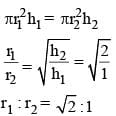SSC CGL Previous Year Questions: Mensuration - 3 - Question 7

A wooden box of dimension 8 metre × 7 metre × 6 metre is to carry rectangular boxes of dimensions 8 cm × 7 cm × 6 cm.
The maximum number of boxes that can be carried in 1 wooden box is    (SSC Sub. Ins. 2014)

Detailed Solution for SSC CGL Previous Year Questions: Mensuration - 3 - Question 7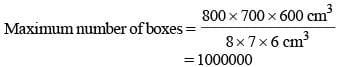SSC CGL Previous Year Questions: Mensuration - 3 - Question 8

The radius of a right circular cone is 3 cm and its height is 4 cm. The total surface area of the cone  is    (SSC Sub. Ins. 2014)

Detailed Solution for SSC CGL Previous Year Questions: Mensuration - 3 - Question 8

Total surface are of cone = πr(l + r)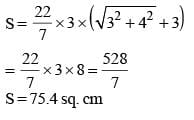SSC CGL Previous Year Questions: Mensuration - 3 - Question 9

A spherical ball of lead of radius 14 cm is melted and recast into spheres of radius 2 cm. The number of the small spheres is   (SSC Multitasking 2014)

Detailed Solution for SSC CGL Previous Year Questions: Mensuration - 3 - Question 9

Number of small spheres,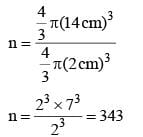SSC CGL Previous Year Questions: Mensuration - 3 - Question 10

Length of each equal side of an isosceles triangle is 10 cm and the included angle between those two sides is 45°. Find the area of the triangle.    (SSC Multitasking 2014)

Detailed Solution for SSC CGL Previous Year Questions: Mensuration - 3 - Question 10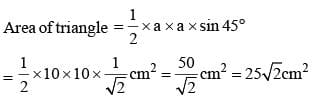SSC CGL Previous Year Questions: Mensuration - 3 - Question 11

The length and breadth of a rectangle are 20 m and 15 m respectively. If length is increased by 20% and the breadth by 30%, the percentage increase in its area is    (SSC Multitasking 2014)

Detailed Solution for SSC CGL Previous Year Questions: Mensuration - 3 - Question 11

Area of rectangle, A = 20 m × 15 m = 300m2.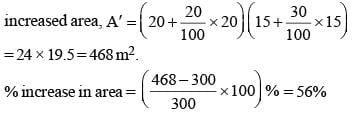SSC CGL Previous Year Questions: Mensuration - 3 - Question 12

A sphere of diameter 6 cm is dropped in a right circular cylindrical vessel partly filled with water. The diameter of the Cylindrical vessel is 12 cm.  If the sphere is just completely submerged in water, then the rise of water level in the cylindrical vessel is   (SSC CGL 1st Sit. 2013)

Detailed Solution for SSC CGL Previous Year Questions: Mensuration - 3 - Question 12

Volume of sphere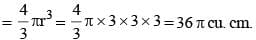If the water level rises by h cm, then
πR2h = 36p
⇒ 6 × 6 × h = 36
⇒ h = 1 cm

SSC CGL Previous Year Questions: Mensuration - 3 - Question 13

The perimeter of the base of a right circular cylinder is 'a' unit. if the volume of the cylinder is V cubic unit. then the height of the cylinder is    (SSC CGL 1st Sit. 2013)

Detailed Solution for SSC CGL Previous Year Questions: Mensuration - 3 - Question 13

If the radius of base of cylinder be r units and its height be h units, then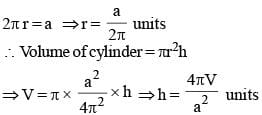SSC CGL Previous Year Questions: Mensuration - 3 - Question 14

The difference of perimeter and diameter of a circle is X unit. The diameter of the circle is    (SSC CGL 1st Sit. 2013)

Detailed Solution for SSC CGL Previous Year Questions: Mensuration - 3 - Question 14

If the diameter of the circle be d units, then
πd – d = X
⇒ d (π – 1) = X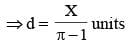SSC CGL Previous Year Questions: Mensuration - 3 - Question 15

If the diagonal of a square is doubled, then its area will be   (SSC CGL 1st Sit. 2013)

Detailed Solution for SSC CGL Previous Year Questions: Mensuration - 3 - Question 15

Diagonal of a square (d) = √2 × side of square (a).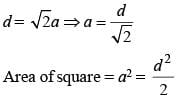Now, diagonal gests doubled, then side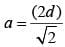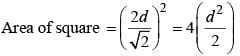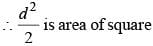Therefore, area will he four times.

SSC CGL Previous Year Questions: Mensuration - 3 - Question 16

The biggest possible circle is inscribed in a rectangle of length 16 cm and breadth 6 cm. Then its area is   (SSC CGL 1st Sit. 2013)

Detailed Solution for SSC CGL Previous Year Questions: Mensuration - 3 - Question 16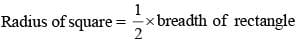= 6/2 = 3 cm
∴ The area of circle = πr2 = 9πcm2.

SSC CGL Previous Year Questions: Mensuration - 3 - Question 17

A square is inscribed in a circle of radius 8 cm. The area of the square is    (SSC CGL 1st Sit. 2013)

Detailed Solution for SSC CGL Previous Year Questions: Mensuration - 3 - Question 17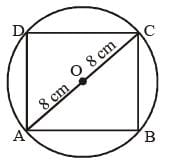Diagonal of square = Diameter of circle
√2 x side of square = 16 cm
Squaring on both sides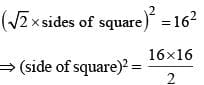⇒ Area of square = 128 sq. cm.

SSC CGL Previous Year Questions: Mensuration - 3 - Question 18

A conical flask is full of water. The flask has base radius r and height h. This water is poured into a cylindrical flask of base radius mr. The height of water in the cylindrical flask is   (SSC CGL 1st Sit. 2013)

Detailed Solution for SSC CGL Previous Year Questions: Mensuration - 3 - Question 18

Volume of water in conical flask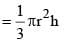If the height of water level in cylindrical flask be H units, then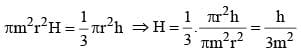SSC CGL Previous Year Questions: Mensuration - 3 - Question 19

The total surface area of a sphere is 8 p square unit. The volume of the sphere is    (SSC CGL 1st Sit. 2013)

Detailed Solution for SSC CGL Previous Year Questions: Mensuration - 3 - Question 19

Surface area of sphere = 4πr2
⇒ 4πr2 = 8π
⇒ r2 = 2 ⇒ r ⇒ √2 units
∴ Volume of sphere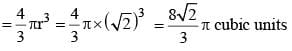SSC CGL Previous Year Questions: Mensuration - 3 - Question 20

The diameters of two circles are the side of a square and the diagonal of the square. The ratio of the areas of the smaller circle and the larger circle is   (SSC CGL 1st Sit. 2013)

Detailed Solution for SSC CGL Previous Year Questions: Mensuration - 3 - Question 20

Side of square = x units
Diagonal of square = √2x units
Radius of smaller circle = x/2 units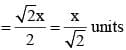∴ Required ratio of areas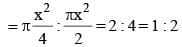SSC CGL Previous Year Questions: Mensuration - 3 - Question 21

A horse is tied to a post by a rope. If the horse moves along a circular path always keeping the rope stretched and describes 88 metres when it has traced out 72° at the centre the length of the rope is (Take π = 22/7)    (SSC CGL 2nd Sit. 2013)

Detailed Solution for SSC CGL Previous Year Questions: Mensuration - 3 - Question 21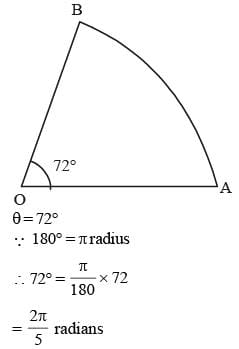Arc AB = s = 88 metre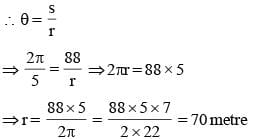SSC CGL Previous Year Questions: Mensuration - 3 - Question 22

If the perimeters of a rectangle and a square are equal and the ratio of two adjacent sides of the rectangle is 1 : 2 then the ratio of area of the rectangle and that of the square is    (SSC CGL 2nd Sit. 2013)

Detailed Solution for SSC CGL Previous Year Questions: Mensuration - 3 - Question 22

Sides of rectangle are 2x and x units.
Side of square = y units
∴ 4y = 6x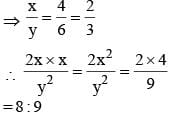SSC CGL Previous Year Questions: Mensuration - 3 - Question 23

A square ABCD is in scribed in a circle of unit radius. Semicircles are described on each side as a diameter. The area of the region bounded by the four semicircles and the circle is    (SSC CGL 2nd Sit. 2013)

Detailed Solution for SSC CGL Previous Year Questions: Mensuration - 3 - Question 23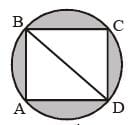BD = 2 units
AB = √2 units
Area of square = 2 square units
Area of four semicircles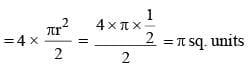∴ Required area = 2 + π – π = 2 sq. units.

SSC CGL Previous Year Questions: Mensuration - 3 - Question 24

The base of a right prism is a triangle whose perimeter is 28 cm and the inradius of the triangle is 4 cm. If the volume of the prism is 366 cc, then its height is    (SSC CHSL 2013)

Detailed Solution for SSC CGL Previous Year Questions: Mensuration - 3 - Question 24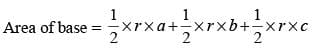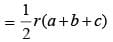=  r x s = 4 x 14= 56 cm2
[Where r = inradius, s = semi-perimeter]
volume of prism = area of base × height
366 = 56 × h
h = 6.5 cm [approx]

SSC CGL Previous Year Questions: Mensuration - 3 - Question 25

The length and breadth of a rectangle are doubled. Percentage increase in area is   (SSC CHSL 2013)

Detailed Solution for SSC CGL Previous Year Questions: Mensuration - 3 - Question 25

A = lb
A' = (2l)(2b) = 4lb = 4A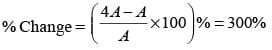SSC CGL Previous Year Questions: Mensuration - 3 - Question 26

If the total surface area of a cube is 96 cm2, its volume is    (SSC CHSL 2013)

Detailed Solution for SSC CGL Previous Year Questions: Mensuration - 3 - Question 26

Total surface area of cube = 96 cm2
6a2 = 96 cm2
a2 = 16 cm2 ⇒ a = 4 cm
Now, volume of cube = a3 = (4)3 = 64 cm3

SSC CGL Previous Year Questions: Mensuration - 3 - Question 27

The volume of air in a room is 204 m3. The height of the room is 6 m. What is the floor area of the room?   (SSC CHSL 2013)

Detailed Solution for SSC CGL Previous Year Questions: Mensuration - 3 - Question 27

Volume of air in room = 204 m3
Area of floor × height of room = 204 m3
Area of floor ×6 = 204 m3
∴ Area of floor = 204/6 = 34 m2

SSC CGL Previous Year Questions: Mensuration - 3 - Question 28

What is the height of a cylinder that has the same volume and radius as a sphere of diameter 12 cm?    (SSC CHSL 2013)

Detailed Solution for SSC CGL Previous Year Questions: Mensuration - 3 - Question 28

Volume of cylinder = volume of sphere (Given)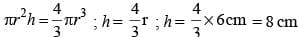SSC CGL Previous Year Questions: Mensuration - 3 - Question 29

The sides of a triangle are 16 cm, 12 cm and 20 cm. Find the area (SSC CHSL 2013)

Detailed Solution for SSC CGL Previous Year Questions: Mensuration - 3 - Question 29

(16)2 + (12)2 = 400 = (20)2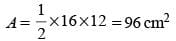SSC CGL Previous Year Questions: Mensuration - 3 - Question 30

A square of side 3 cm is cut off from each corner of a rectangular sheet of length 24 cm and breadth 18 cm and the remaining sheet is folded to form an open rectangular box. The surface area of the box is    (SSC CHSL 2013)

Detailed Solution for SSC CGL Previous Year Questions: Mensuration - 3 - Question 30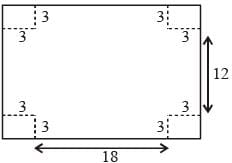l = 18 cm, b = 12 cm, h = 3 cm
S = 2(lh + bh) + lb  {Box is open from upper side}
= 2 (54 + 36) + 216 = 396 cm2

SSC CGL Previous Year Questions: Mensuration - 3 - Question 31

The volumes of a cylinder and a cone are in the ratio 3 : 1. Find their diameters and then compare them when their heights are equal.    (SSC CHSL 2013)

Detailed Solution for SSC CGL Previous Year Questions: Mensuration - 3 - Question 31

Volume of cylinder = 3 × volume of cone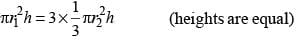r1 = r2
d1 = d2

SSC CGL Previous Year Questions: Mensuration - 3 - Question 32

The base of a right pyramid is an equilateral triangle of side 10√3 cm. If the total surface area of the pyramid is 270√3 sq. cm, its height is    (SSC CHSL 2013)

Detailed Solution for SSC CGL Previous Year Questions: Mensuration - 3 - Question 32

Now, T.S.A of pyramid = ar (ΔABD) + ar (ΔADC) + ar (ΔABC) + ar (ΔBDC)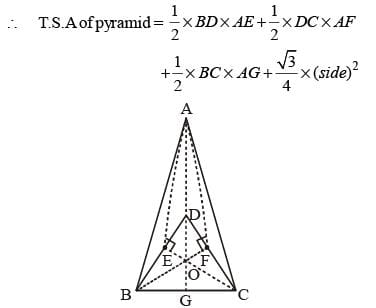(∴ AE = AF = AG = height of isosceles Δ (h))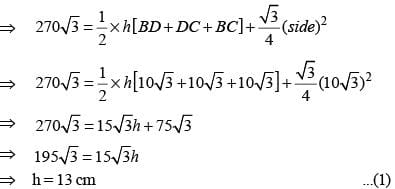Now to find height of pyramid (H), we use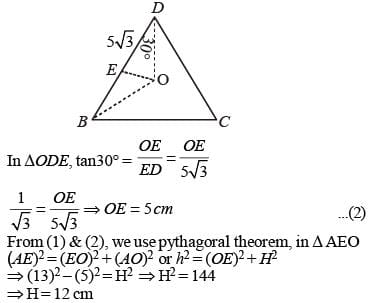SSC CGL Previous Year Questions: Mensuration - 3 - Question 33

If the volumes of two right cir-cular cones are in the ratio 4 : 1 and their diameters are in the ratio 5 : 4 then the ratio of their heights is:    (SSC Sub. Ins. 2013)

Detailed Solution for SSC CGL Previous Year Questions: Mensuration - 3 - Question 33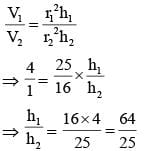SSC CGL Previous Year Questions: Mensuration - 3 - Question 34

A godown is 15 m long and 12 m broad. The sum of the areas of the floor and the ceiling is equal to the sum of areas of the four walls. The volume (in m3) of the godown is:   (SSC Sub. Ins. 2013)

Detailed Solution for SSC CGL Previous Year Questions: Mensuration - 3 - Question 34

If the height of the godown be h meter, then
2(15 × 12) = 2 × h(15 + 12)
⇒ 27h = 15 × 12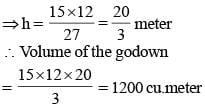SSC CGL Previous Year Questions: Mensuration - 3 - Question 35

Three circles of equal radius 'a' cm touch each other. The area of the shaded region is:    (SSC Sub. Ins. 2013)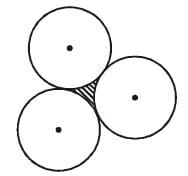Detailed Solution for SSC CGL Previous Year Questions: Mensuration - 3 - Question 35

AB = BC = CA = 2a cm.
∠BAC = ∠ACB = ∠ABC = 60°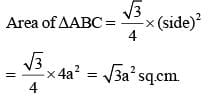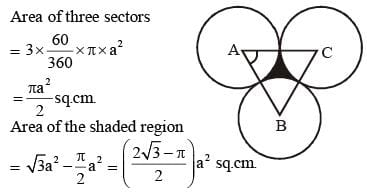SSC CGL Previous Year Questions: Mensuration - 3 - Question 36

Water flows at the rate of 10 metres per minute from a cylindrical pipe 5 mm in diameter. How long it lake to fill up a conical vessel whose diameter at the base is 30 cm and depth 24 cm?    (SSC Sub. Ins. 2013)

Detailed Solution for SSC CGL Previous Year Questions: Mensuration - 3 - Question 36

Volume of water flowing from the pipe in 1 minute
= π x 0.25 x 0.25 x 1000 cu.cm.
Volume of conical vessel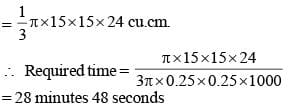SSC CGL Previous Year Questions: Mensuration - 3 - Question 37

A copper sphere of diameter 18 cm is drawn into a wire of diameter 4 mm. The length of the wire, in metre, is:    (SSC Sub. Ins. 2013)

Detailed Solution for SSC CGL Previous Year Questions: Mensuration - 3 - Question 37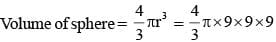= 972 π cubic.cm.
If the length of wire be h cm., then
π × (0.2)2 × h = 972π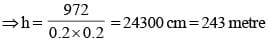SSC CGL Previous Year Questions: Mensuration - 3 - Question 38

If area of an equilateral triangle is a and height b, then value of  b2/a  is:    (SSC Sub. Ins. 2013)

Detailed Solution for SSC CGL Previous Year Questions: Mensuration - 3 - Question 38

Let side of triangle = x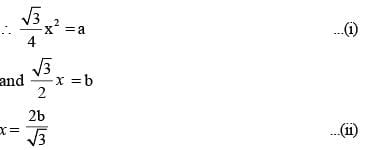Putting x in equation (i)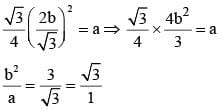SSC CGL Previous Year Questions: Mensuration - 3 - Question 39

A solid right circular cylinder and a solid hemisphere stand on equal bases and have the same height. The ratio of their whole surface areas is:   (SSC Sub. Ins. 2013)

Detailed Solution for SSC CGL Previous Year Questions: Mensuration - 3 - Question 39

Radius of cylinder = r units and height = r units
∴ Surface area of cylinder = 2πr2 + 2πr2 = 4πr2
Surface area of hemisphere = 2πr2 + πr2 = 3πr2
= 4 : 3

SSC CGL Previous Year Questions: Mensuration - 3 - Question 40

If the sum of the length, breadth and height of a rectangular parallelopiped is 24 cm and the length of its diagonal is 15 cm, then its total surface area is    (SSC Multitasking 2013)

Detailed Solution for SSC CGL Previous Year Questions: Mensuration - 3 - Question 40

Let length = l, breadth = b, height = h.
l + b + h = 24 (given) ... (i)
Diagonal of parallellopiped = 15 cm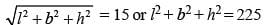Squaring eqn. (i) on both sides
l2 + b2 + h2 + 2lb + 2bh + 2hl = 576
2(lb + bh + hl) = 576 – 225 = 351
[∵ Surface area of parallellopiped = 2(lb + bh + hl)]

## SSC CGL (Tier - 1) - Previous Year Papers (Topic Wise)

250 tests
Information about SSC CGL Previous Year Questions: Mensuration - 3 Page
In this test you can find the Exam questions for SSC CGL Previous Year Questions: Mensuration - 3 solved & explained in the simplest way possible. Besides giving Questions and answers for SSC CGL Previous Year Questions: Mensuration - 3, EduRev gives you an ample number of Online tests for practice

250 tests